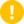## Name

ST_Disjoint — Tests if two geometries have no points in common

## Synopsis

`boolean ST_Disjoint(` geometry A , geometry B `)`;

## Description

Returns `true` if two geometries are disjoint. Geometries are disjoint if they have no point in common.

If any other spatial relationship is true for a pair of geometries, they are not disjoint. Disjoint implies that ST_Intersects is false.

In mathematical terms: ST_Disjoint(A, B) ⇔ A ⋂ B = ∅Enhanced: 3.0.0 enabled support for `GEOMETRYCOLLECTION`

Performed by the GEOS moduleThis function call does not use indexes. A negated ST_Intersects predicate can be used as a more performant alternative that uses indexes: `ST_Disjoint(A,B) = NOT ST_Intersects(A,B)`NOTE: this is the "allowable" version that returns a boolean, not an integer.This method implements the OGC Simple Features Implementation Specification for SQL 1.1.

s2.1.1.2 //s2.1.13.3 - a.Relate(b, 'FF*FF****')This method implements the SQL/MM specification.

SQL-MM 3: 5.1.26

## Examples

```SELECT ST_Disjoint('POINT(0 0)'::geometry, 'LINESTRING ( 2 0, 0 2 )'::geometry);
st_disjoint
---------------
t
(1 row)
SELECT ST_Disjoint('POINT(0 0)'::geometry, 'LINESTRING ( 0 0, 0 2 )'::geometry);
st_disjoint
---------------
f
(1 row)
```